# OpenCV | Hands on Image Contrast

Contrast means to change the value of each and every image pixel. This change can be done by either multiplying or dividing the pixel values of the image, by any constant. This article gives an in-depth knowledge about how can an image contrast be changed using OpenCV.

```Input :
Original Image

Output :
-> Original Image
-> Image with contrast increased by 4
-> Image with contrast increased by 2
-> Image with contrast decreased by .5
-> Image with contrast decreased by .25

```

Code : CPP code to increase or decrease the contrast of an iamge

 `// c++ code explaining how to ` `// increase or decrease the  ` `// contrast of an image ` ` `  `// loading library files ` `#include ` `#include ` `#include ` ` `  `using` `namespace` `cv; ` `using` `namespace` `std; ` ` `  `int` `main(``int` `argc, ``char``** argv) ` `{ ` `    ``// Loading the Image File under testing ` `    ``Mat image = imread(``"C:\\Users\\dell\\Desktop\\abc.jpg"``); ` ` `  `    ``// Check whether the image is present or not ` `    ``if` `(image.empty()) { ` `        ``cout << ``"Could not open or find the image"` `<< endl; ` ` `  `        ``// waiting for any  key to be pressed ` `        ``return` `-1; ` `    ``} ` ` `  `    ``// Declaring the Contrast instances ` ` `  `    ``// increasing the contrast level by 100% ` `    ``Mat imageContrastHigh2; ` `    ``image.convertTo(imageContrastHigh2, -1, 2, 0); ` ` `  `    ``// increasing the contrast level by 200% ` `    ``Mat imageContrastHigh4; ` `    ``image.convertTo(imageContrastHigh4, -1, 4, 0); ` ` `  `    ``// decreasing the contrast level by 50% ` `    ``Mat imageContrastLow0_5; ` `    ``image.convertTo(imageContrastLow0_5, -1, 0.5, 0); ` ` `  `    ``// decreasing the contrast level by 75% ` `    ``Mat imageContrastLow0_25; ` `    ``image.convertTo(imageContrastLow0_25, -1, 0.25, 0); ` ` `  `    ``// Declaring the windows ` `    ``// for images belonging to different contrast level ` `    ``String windowNameOriginalImage = ``"Original Image"``; ` `    ``String windowNameContrastHigh2 = ``"Contrast Increased by 2"``; ` `    ``String windowNameContrastHigh4 = ``"Contrast Increased by 4"``; ` `    ``String windowNameContrastLow0_5 = ``"Contrast Decreased by 0.5"``; ` `    ``String windowNameContrastLow0_25 = ``"Contrast Decreased by 0.25"``; ` ` `  `    ``// Running the window instance ` `    ``// and opening it ` `    ``namedWindow(windowNameOriginalImage, WINDOW_NORMAL); ` `    ``namedWindow(windowNameContrastHigh2, WINDOW_NORMAL); ` `    ``namedWindow(windowNameContrastHigh4, WINDOW_NORMAL); ` `    ``namedWindow(windowNameContrastLow0_5, WINDOW_NORMAL); ` `    ``namedWindow(windowNameContrastLow0_25, WINDOW_NORMAL); ` ` `  `    ``// Loading images inside the above created Windows ` `    ``imshow(windowNameOriginalImage, image); ` `    ``imshow(windowNameContrastHigh2, imageContrastHigh2); ` `    ``imshow(windowNameContrastHigh4, imageContrastHigh4); ` `    ``imshow(windowNameContrastLow0_5, imageContrastLow0_5); ` `    ``imshow(windowNameContrastLow0_25, imageContrastLow0_25); ` ` `  `    ``// waiting for any key to be pressed ` `    ``waitKey(0); ` ` `  `    ``// closing all the windows instances ` `    ``// when any key is pressed. ` `    ``destroyAllWindows(); ` ` `  `    ``return` `0; ` `} `

Input :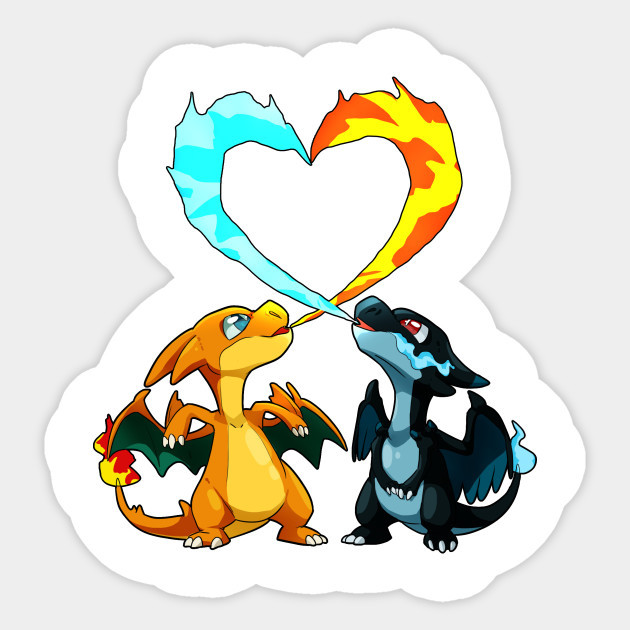Output :

Image with contrast increased by 4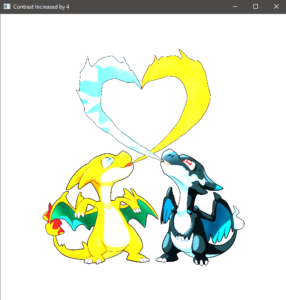Image with contrast increased by 2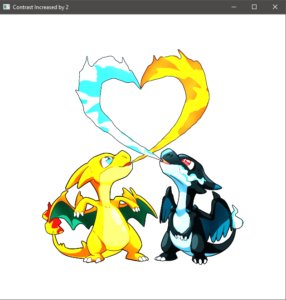Image with contrast decreased by .5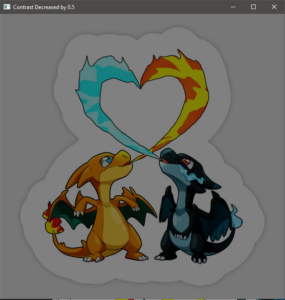Image with contrast decreased by .25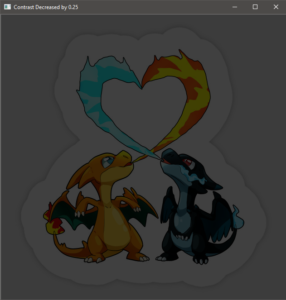## Explanation :

 `// Declaring the Contrast instances ` ` `  `// increasing the contrast level by 100% ` `Mat imageContrastHigh2; ` `image.convertTo(imageContrastHigh2, -1, 2, 0); ` ` `  `// increasing the contrast level by 200% ` `Mat imageContrastHigh4; ` `image.convertTo(imageContrastHigh4, -1, 4, 0); ` ` `  `// decreasing the contrast level by 50% ` `Mat imageContrastLow0_5; ` `image.convertTo(imageContrastLow0_5, -1, 0.5, 0); ` ` `  `// decreasing the contrast level by 75% ` `Mat imageContrastLow0_25; ` `image.convertTo(imageContrastLow0_25, -1, 0.25, 0); `

These code lines will change the image pixel values by a specified amount. The final changed image is then stored to the given output image. The contrast level will increase if the specified amount factor is greater than 1, otherwise will decrease if the specified amount factor is less than 1.

MAT Function :
The “MAT” function changes each image pixel value to the target data type and the values are changed as the degree of factor multiplied.

```Parameters :

m      : Output Image
rtype  : Output Image type, Output Image type is same
as input if it is set to -ve value
alpha  : Input image pixel are multiplied with this
number prior to its assignment to output image
beta   : adding this value to each input image pixel
```

My Personal Notes arrow_drop_upCheck out this Author's contributed articles.

If you like GeeksforGeeks and would like to contribute, you can also write an article using contribute.geeksforgeeks.org or mail your article to contribute@geeksforgeeks.org. See your article appearing on the GeeksforGeeks main page and help other Geeks.

Please Improve this article if you find anything incorrect by clicking on the "Improve Article" button below.

Article Tags :
Practice Tags :

Be the First to upvote.

Please write to us at contribute@geeksforgeeks.org to report any issue with the above content.